# Least Common Multiple

Go back to  'Numbers'

 1 Concept Video on LCM 2 Definition of Multiple 3 Definition of Common Multiple 4 Definition of least Common Multiple 5 Methods of finding LCM 6 Least Common Multiple Calculator 7 LCM Formula 8 Thinking out of the Box! 9 Solved Examples on LCM 10 Challenging Questions on LCM 11 Practice Questions on LCM 12 Maths Olympiad Sample Papers 13 Frequently Asked Questions (FAQs) 14 FREE Worksheets of LCM

We at Cuemath believe that Math is a life skill. Our Math Experts focus on the “Why” behind the “What.” Students can explore from a huge range of interactive worksheets, visuals, simulations, practice tests, and more to understand a concept in depth.

Book a FREE trial class today! and experience Cuemath’s LIVE Online Class with your child.

## Understanding LCM (Least Common Multiple)

Before we begin explaining about LCM, let us first get an idea of the concept with the help of this simple video:

## Definition of Multiple

A multiple is a number that is obtained by multiplying the given number by another natural number.

For example:

Let’s consider $$3$$.

Multiples of $$3$$ are obtained by multiplying it by the natural numbers $$1, 2, 3, 4, 5, 6, …$$.

So the multiples of $$3$$ are $$3, 6, 9, 12, 15, 18, ...$$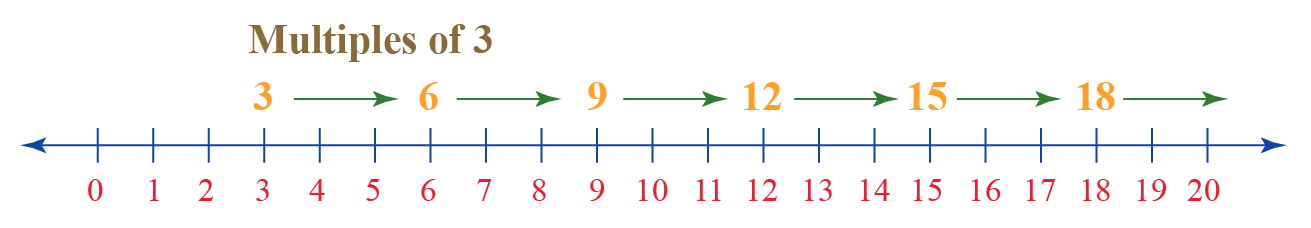✍️Note: A number has an infinite number of multiples.

## Definition of Common Multiple

A common multiple of two numbers is a number that is a multiple of each of the numbers.

Let’s take two numbers, say, $$2$$ and $$5$$.

Each will have its own set of multiples.

• Multiples of $$2$$ are $$2, 4, 6, 8, 10, 12, 14, 16, 18, 20, …$$
• Multiples of $$5$$ are $$5, 10, 15, 20,….$$

Representing these multiples on the number line: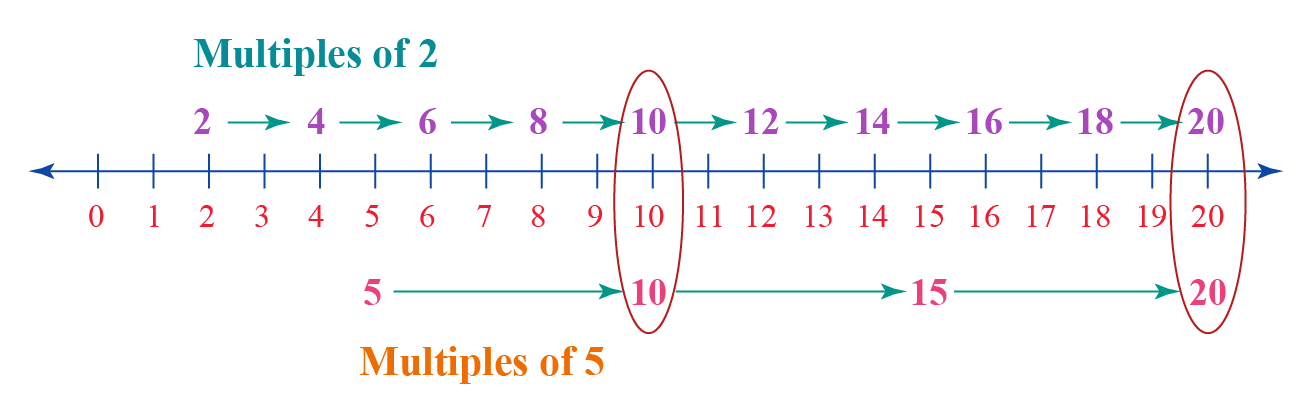Among these, the multiples that are common to each of these numbers are $$10, 20, ,...$$.

These are called the common multiples of $$2$$ and $$5$$.

## Definition of Least Common Multiple (LCM)

The least common multiple of two or more numbers is the smallest number among all common multiples of the given numbers.

In the previous example, the common multiples of $$2$$ and $$5$$ are $$10, 20, …$$.

The smallest number among $$10, 20, …$$ is $$10$$.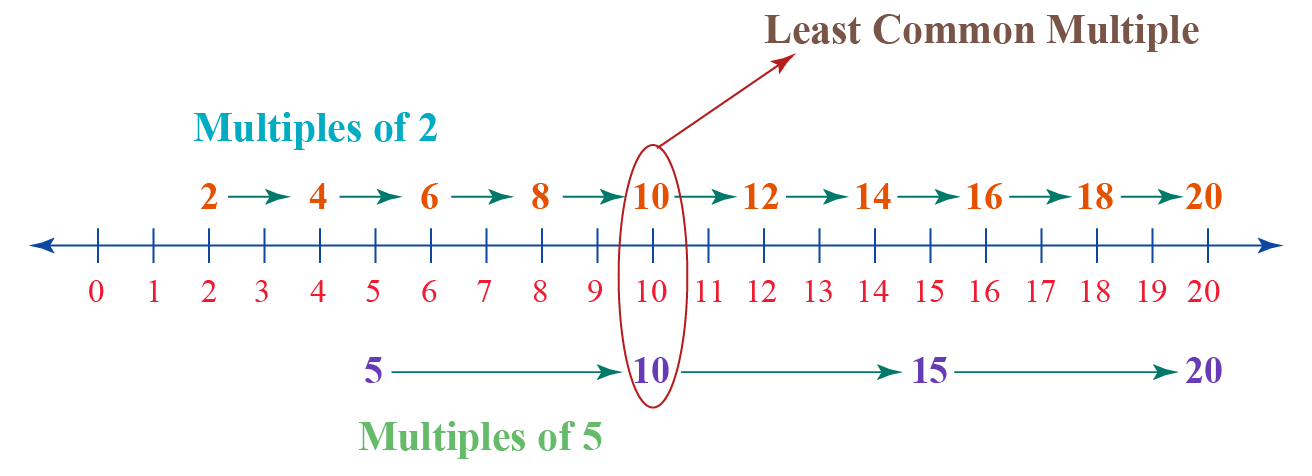So the least common multiple of $$2$$ and $$5$$ is $$10$$.

This can be written as:

 ∴ $$LCM (2, 5) = 10$$

### LCM Examples

Using the above definition, the LCM of

• $$3$$ and $$7$$ is $$21$$, i.e. LCM $$(3,7) = 21$$.
• $$18$$ and $$24$$ is $$72$$, i.e. LCM $$(18,24) = 72$$.
• $$60$$ and $$90$$ is $$180$$, i.e. LCM $$(60,90) = 180$$.
• $$10$$ and $$17$$ is $$170$$, i.e. LCM $$(10,17) = 170$$.

## Methods of finding LCM

There are 3 methods to calculate the LCM of two numbers:

1. by listing out the common multiples
2. by prime factorization
3. by division method

### 1. By listing out the Common Multiples

Let’s learn this method using the example below.

Example:

What is the LCM of $$4$$ & $$5$$?

Solution:

Step 1 - List a few multiples of $$4$$ & $$5$$,

• Multiples of 4 - $$4, 8, 12, 16, 20, 24, 28$$
• Multiples of 5 - $$5, 10, 15, 20, 25$$

Step 2 - Circle all the common multiples.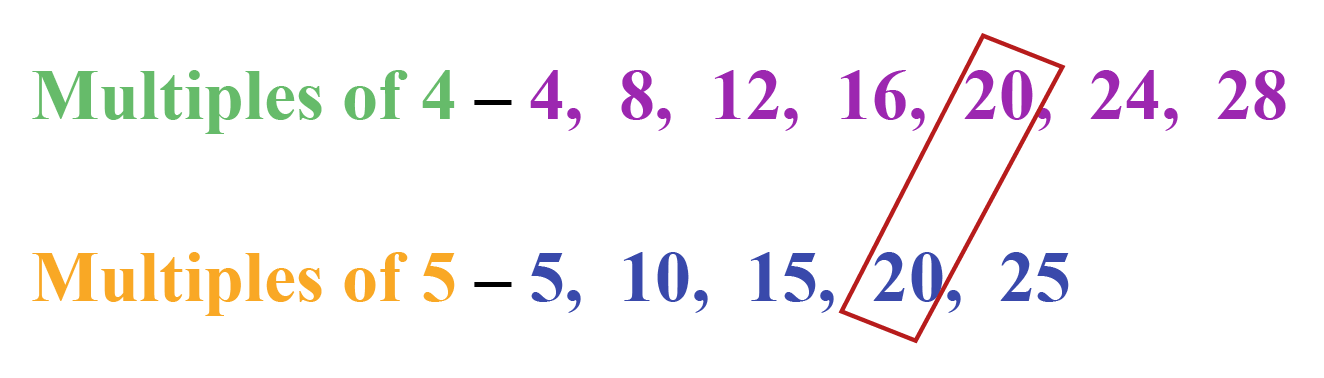Step 3 - $$20$$ is the first (smallest) number that is present in both the lists.

Hence the LCM of $$4$$ & $$5$$ is $$20$$.

 ∴ $$LCM$$ $$of$$ $$4$$ $$and$$ $$5$$ = $$20$$

### 2. By Prime Factorisation

Let’s learn this method using the examples below.

Example 1

What is the LCM of $$60$$ and $$90$$ using the prime factorization?

Solution

Step 1 - Represent the numbers in the prime factored form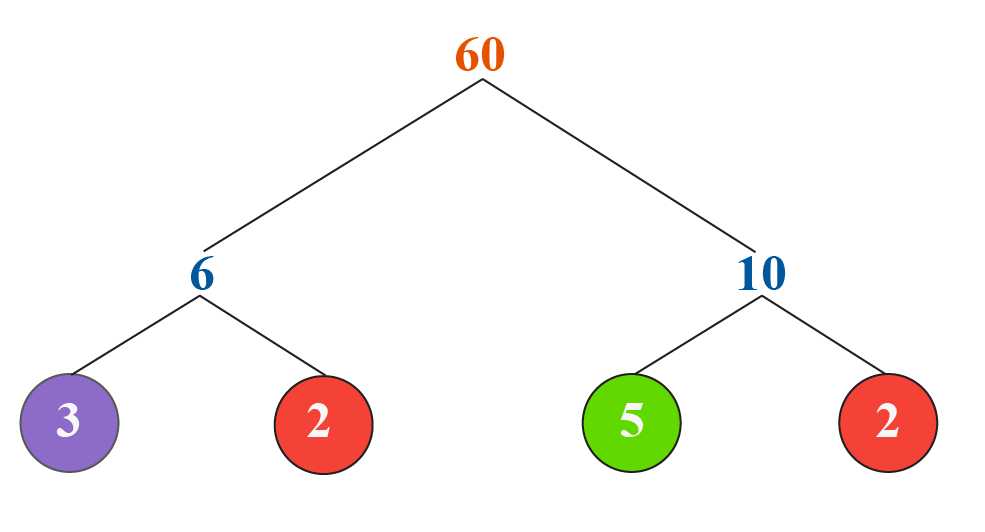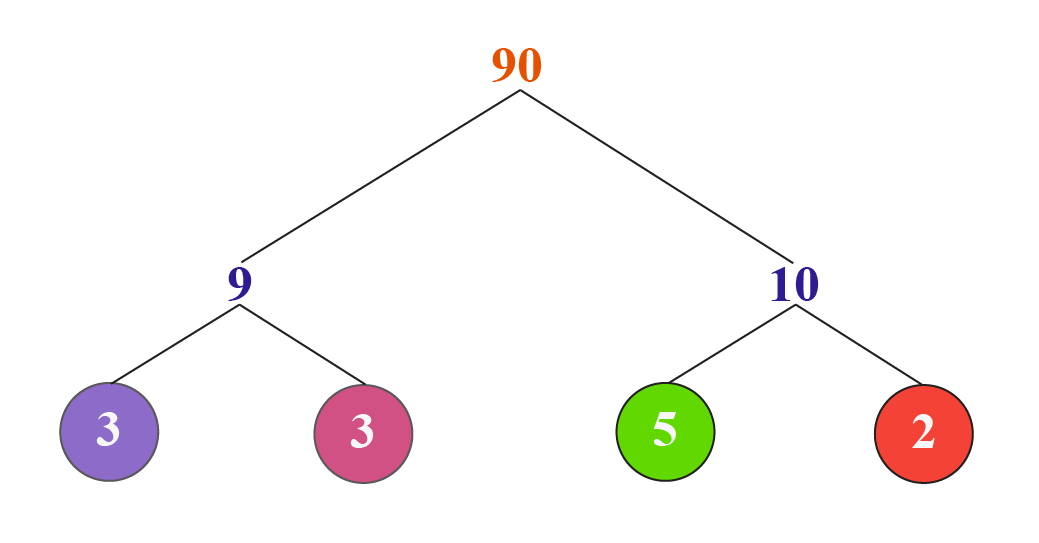\begin{align} 60&= 2 \times 2 \times 3 \times 5\\ 90&=2 \times 3\times 3 \times 5 \end{align}

Step 2 - The LCM of the given two numbers is the product of all the prime factors. However, common factors will be included only once.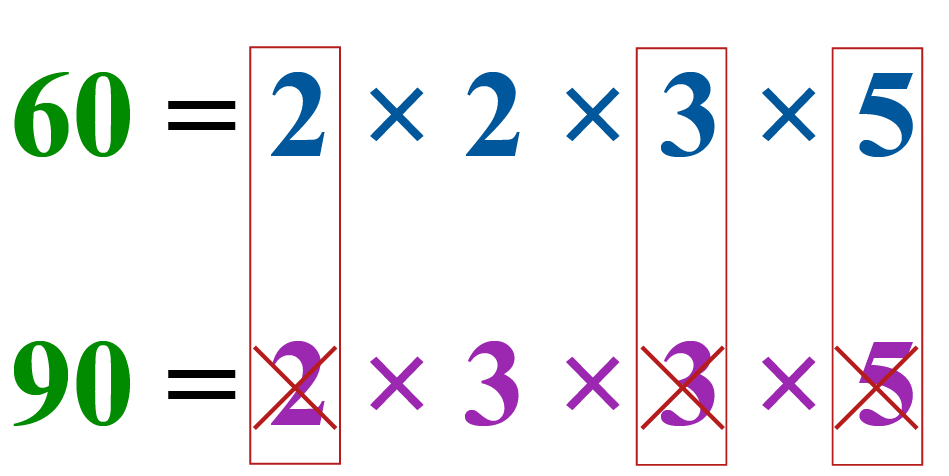Still confusing?? Then let’s see the following Venn diagram to understand this process easier.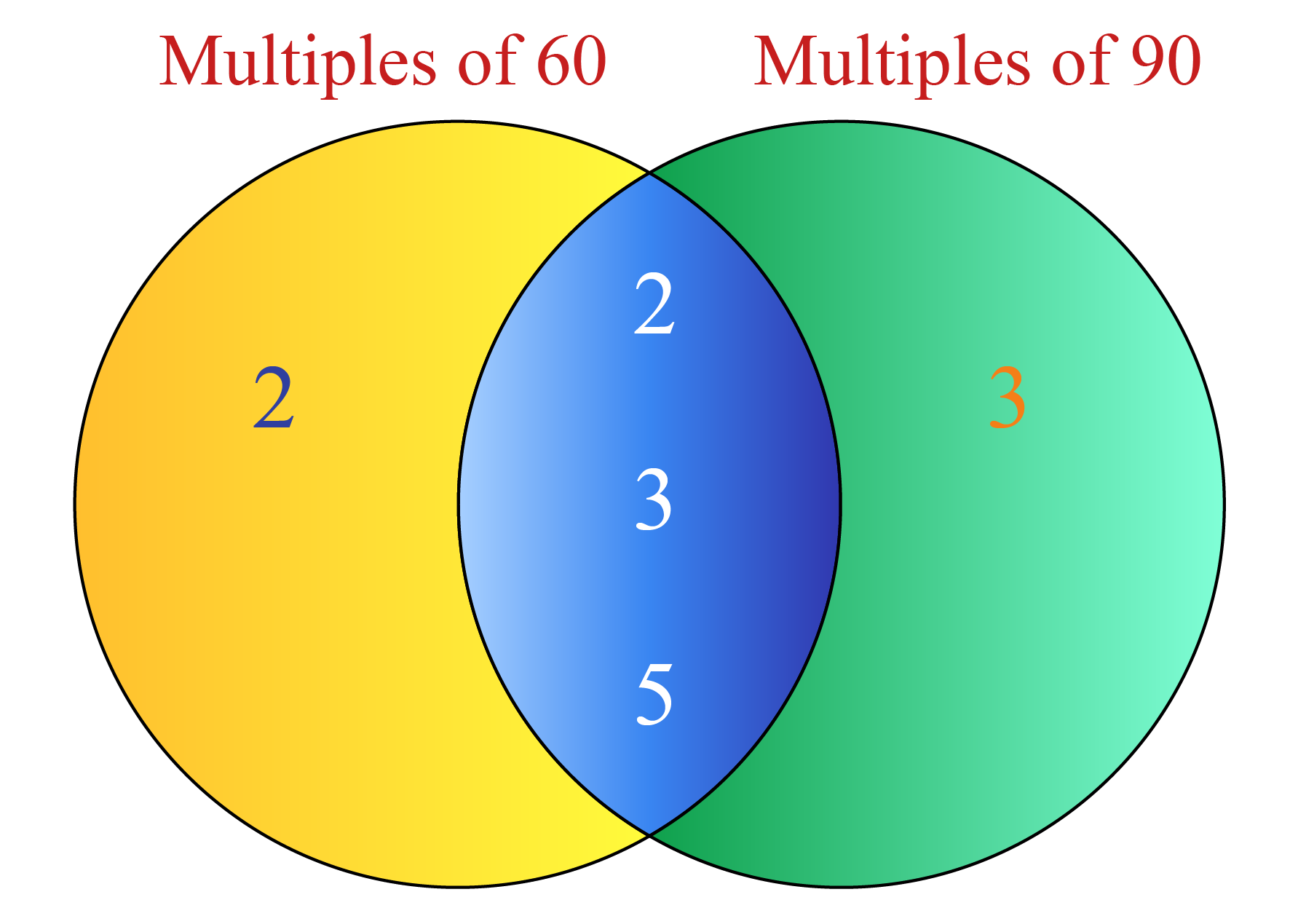Thus, LCM of $$60$$ and $$90$$ = $$2 \times 2 \times 3 \times 5 \times 3$$ = $$180$$.

 ∴ $$LCM$$ $$of$$ $$60$$ $$and$$ $$90$$ = $$180$$

Example 2

What is the LCM of $$24$$ and $$60$$ using the prime factorization?

Solution

Step 1 - Represent the numbers in the prime factored form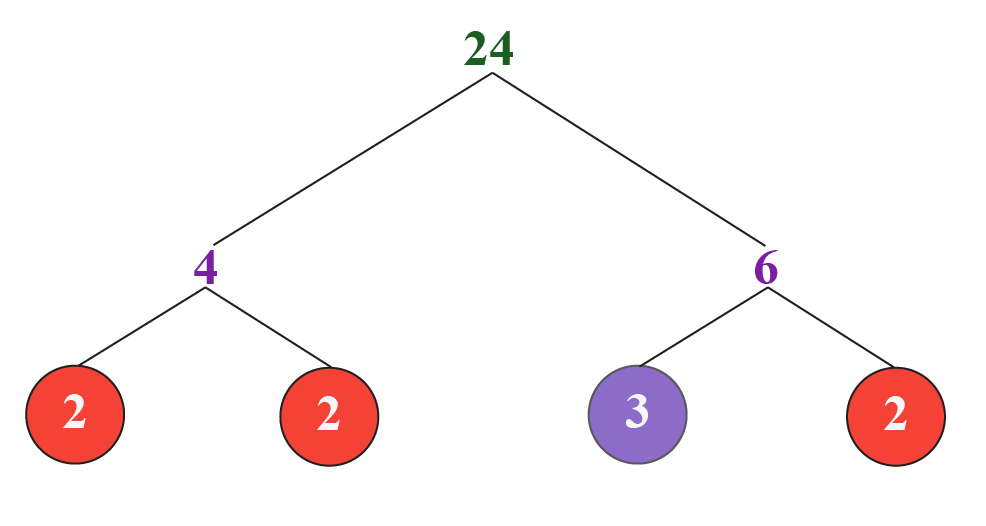Thus,
$\begin{array}{l} 24=2^{3} \times 3^{1} \\ 60=2^{2} \times 3^{1} \times 5^{1} \end{array}$

Step 2 - The LCM of the two numbers will be the product of the greatest power of each prime factor occurring in both the numbers.
$\mathrm{LCM}(24,60)=2^{3} \times 3^{1} \times 5^{1}=120$

 ∴ $$LCM$$ $$of$$ $$24$$ $$and$$ $$60$$ = $$120$$

### 3. Division Method

Let’s learn this method using the example below.

Example

What is the LCM of $$6$$ & $$15$$ using the common division method?

Solution

Step 1 - Find a which is a factor of at least one of the given numbers.

Write this prime number on the left of the two numbers (as shown).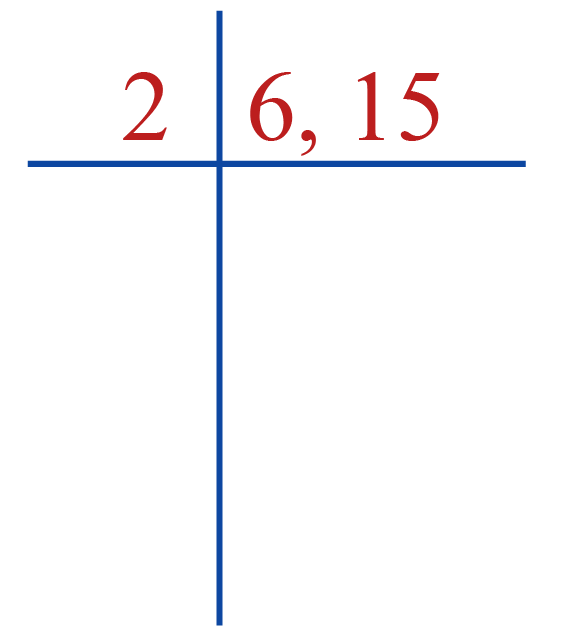For each number in the right column, do the following:

Step 2 - If the prime number in step 1 is a factor of the number, then divide the number by the prime and write the quotient below.

If the prime number in step 1 is not a factor of the number, then write the number in the row below as it is.

Continue the steps until all co-prime numbers are left in the last row.

Two numbers are said to be coprime if they share no common factors other than 1.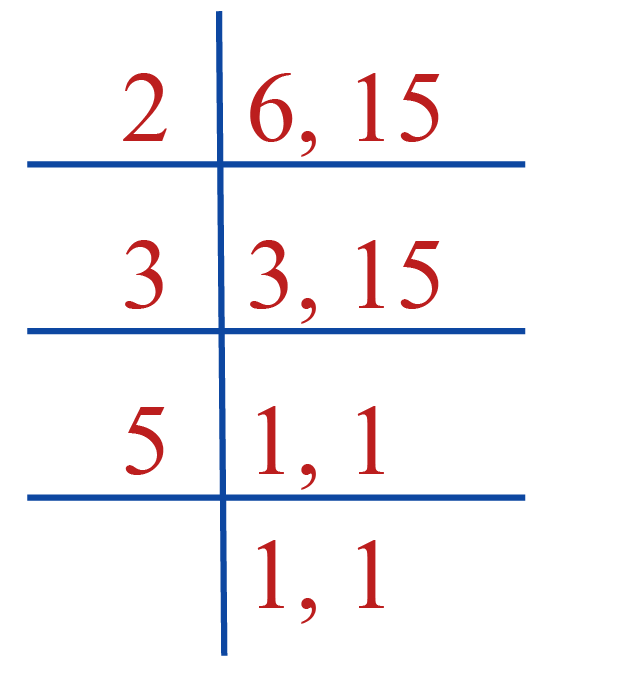Step 3 - The LCM is the product of all the prime numbers.

LCM of $$6$$ and $$15$$ is $$2 \times 3\times 5 = 30$$.

 ∴ $$LCM$$ $$of$$ $$6$$ $$and$$ $$15$$ = $$30$$

Though we have three methods to find the LCM, the prime factorisation method is the most common and easy method that we use.

Help your child score higher with Cuemath’s proprietary FREE Diagnostic Test. Get access to detailed reports, customised learning plans and a FREE counselling session. Attempt the test now.

## Least Common Multiple Calculator

Let’s enter two numbers in the calculator below and it would give the LCM of the given numbers along with a step-by-step procedure using division method.

Using the calculator, we can check our LCM after solving using one of the above three methods.

CLUEless in Math? Check out how CUEMATH Teachers will explain Least Common Multiple to your kid using interactive simulations & worksheets so they never have to memorise anything in Math again!

Explore Cuemath Live, Interactive & Personalised Online Classes to make your kid a Math Expert. Book a FREE trial class today!

## LCM Formula

Let's take any 2 numbers. If we know how to calculate the HCF of these 2 numbers, we can find their LCM using the formula given below:

 $$\text{LCM of two numbers}= \frac{\text{Product of two numbers}}{\text{HCF of two numbers}}$$

Example:
Let’s find the LCM of $$6$$ and $$15$$ using the above formula.

Solution:
We know that the HCF of $$6$$ and $$15$$ is $$3$$.
Using the above formula,
\begin{align} \text{LCM }(6, 15)& = \dfrac{6 \times 15}{\text{HCF } (6, 15)}\\&= \dfrac{90}{3}\\&= 30 \end{align}

 ∴ $$LCM$$ (6, 15)= $$30$$Think Tank
1. How would you find the area of a sector of the circle?
2. To warn ships of underwater rocks, a lighthouse spreads a red-coloured light over a sector of angle $${80^\circ}$$. The light reaches a distance of 16.5 km. Find the area of the sea over which the ships are warned.
3. Tom is riding a cycle to his friend's house. The wheel of his cycle has a radius of 20 cm and his friend lives 660 m away. How many times does his front wheel rotate when he goes and comes back from his friend's house?

## Solved Examples

 Example 1

Find the LCM of $$980$$ and $$9000$$ using the prime factorisation method.

Solution:

First, we carry out the prime factorization of the two numbers: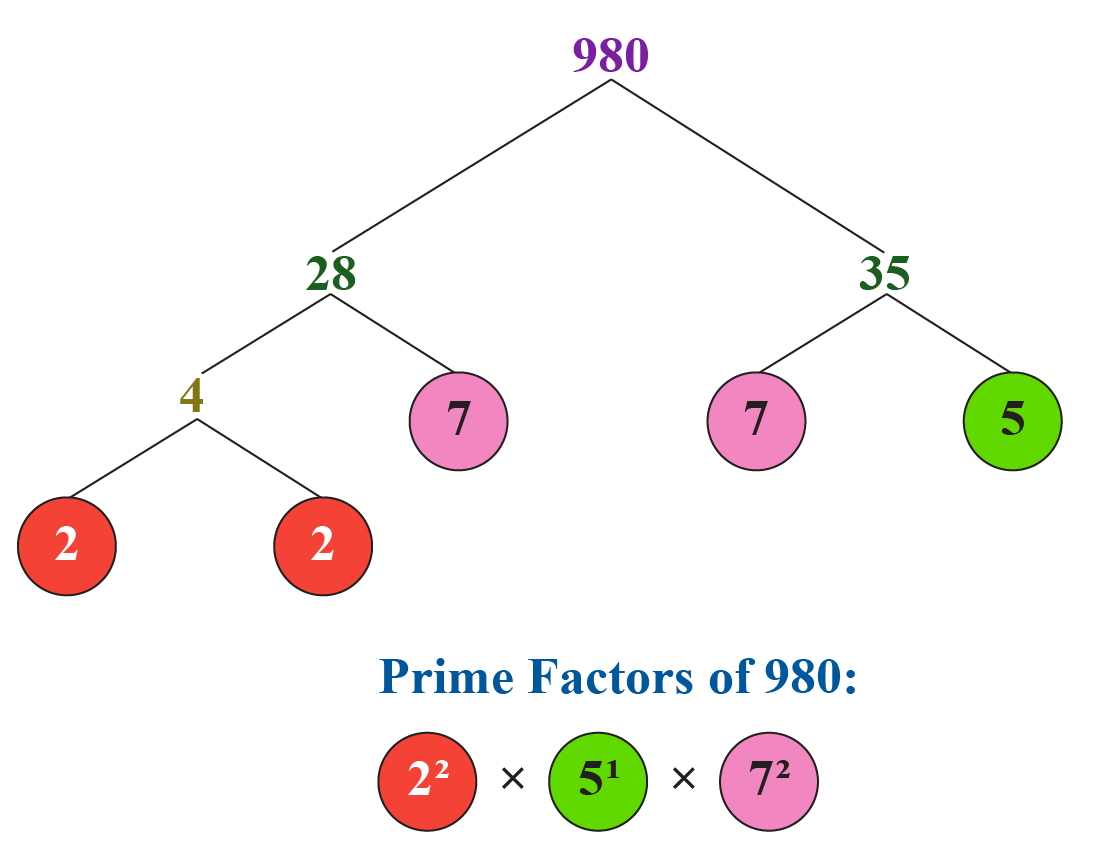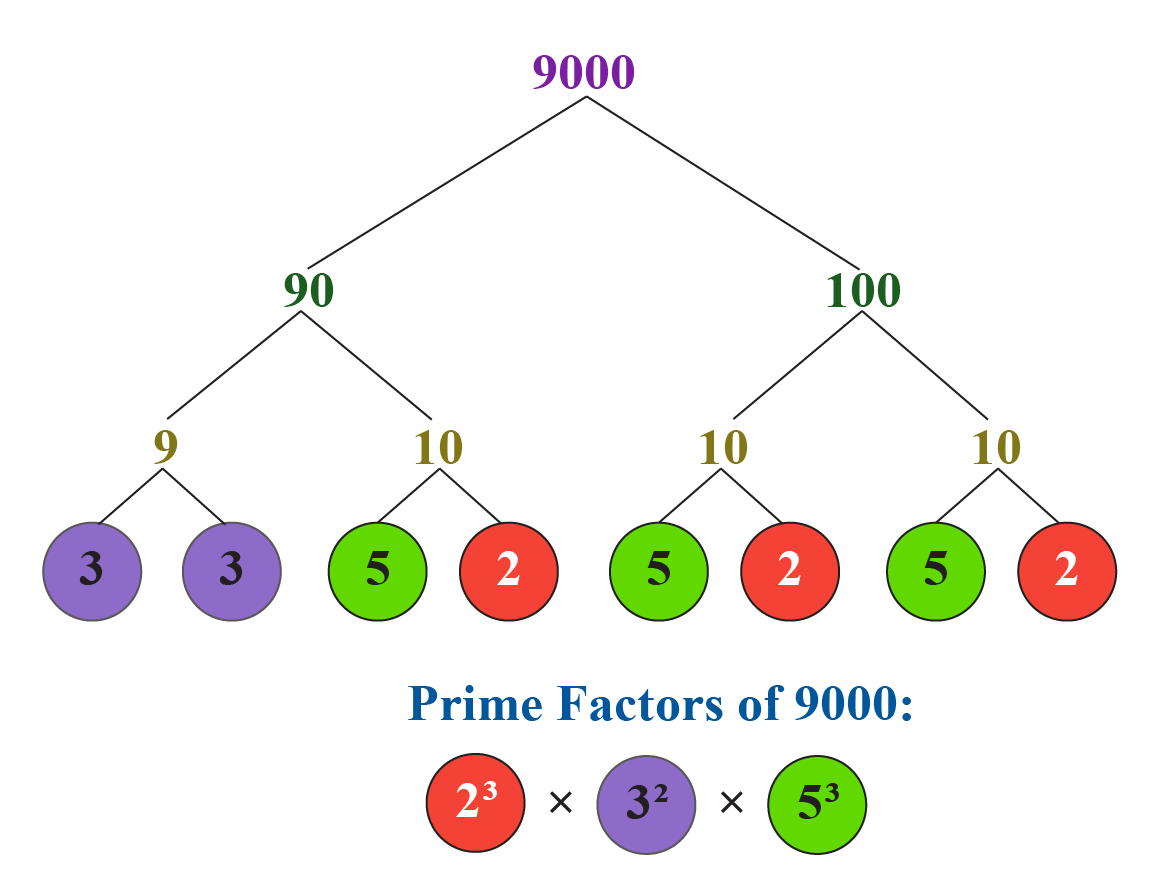The LCM will be the product of the greatest power of each prime factor occurring in the numbers:

\begin{align} {\text{LCM}}\left( {980,\;9000} \right) &= {2^3} \times {3^2} \times {5^3} \times {7^2} \hfill \\ \qquad \qquad \qquad \qquad \quad \; &= {441000} \hfill \\ \end{align}

 ∴ $$LCM$$ (980, 9000)= $$441000$$
 Example 2

Find the LCM of $$371250$$ and $$29040$$ using the prime factorisation method.

Solution:

First, we carry out the prime factorization of the two numbers: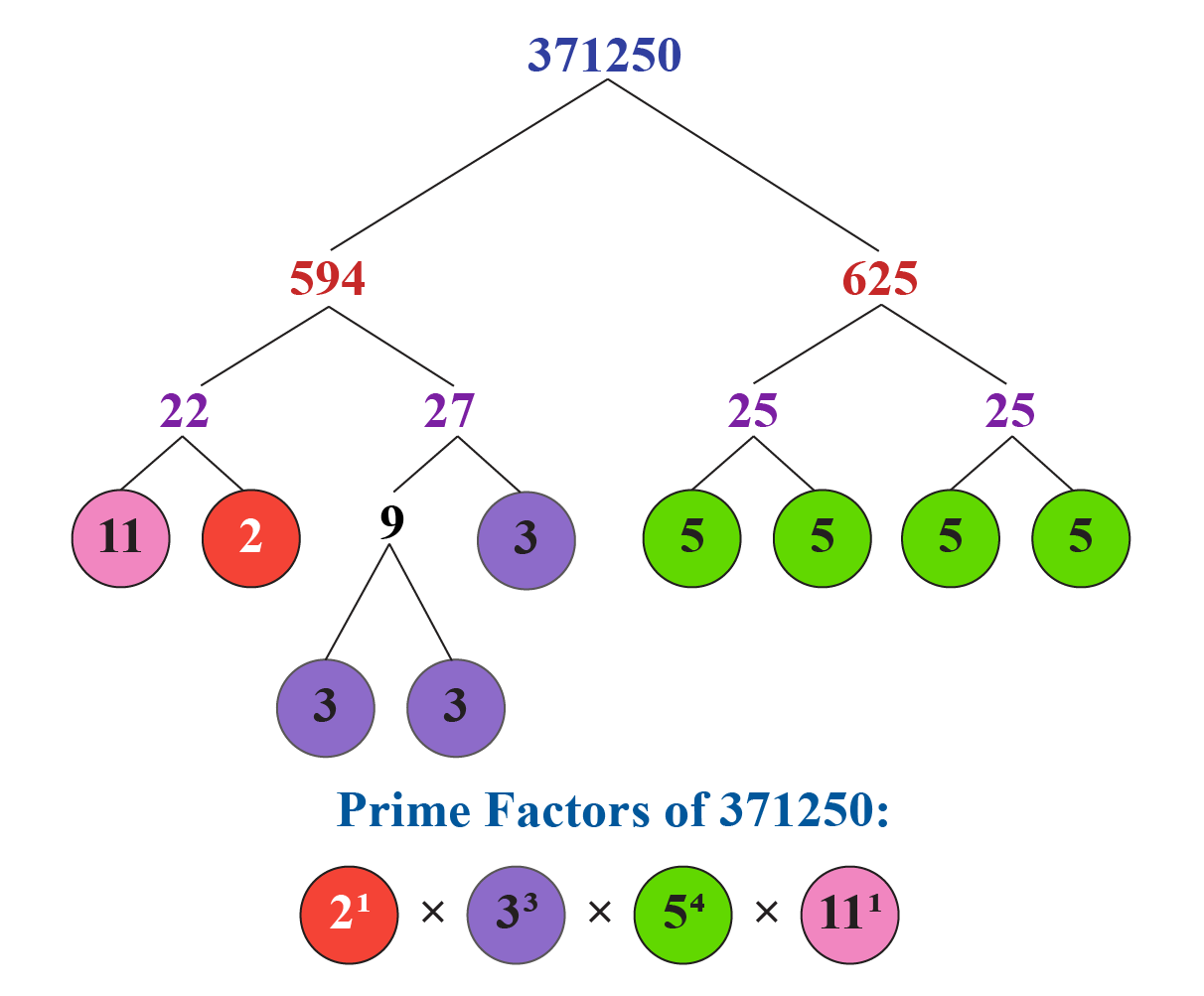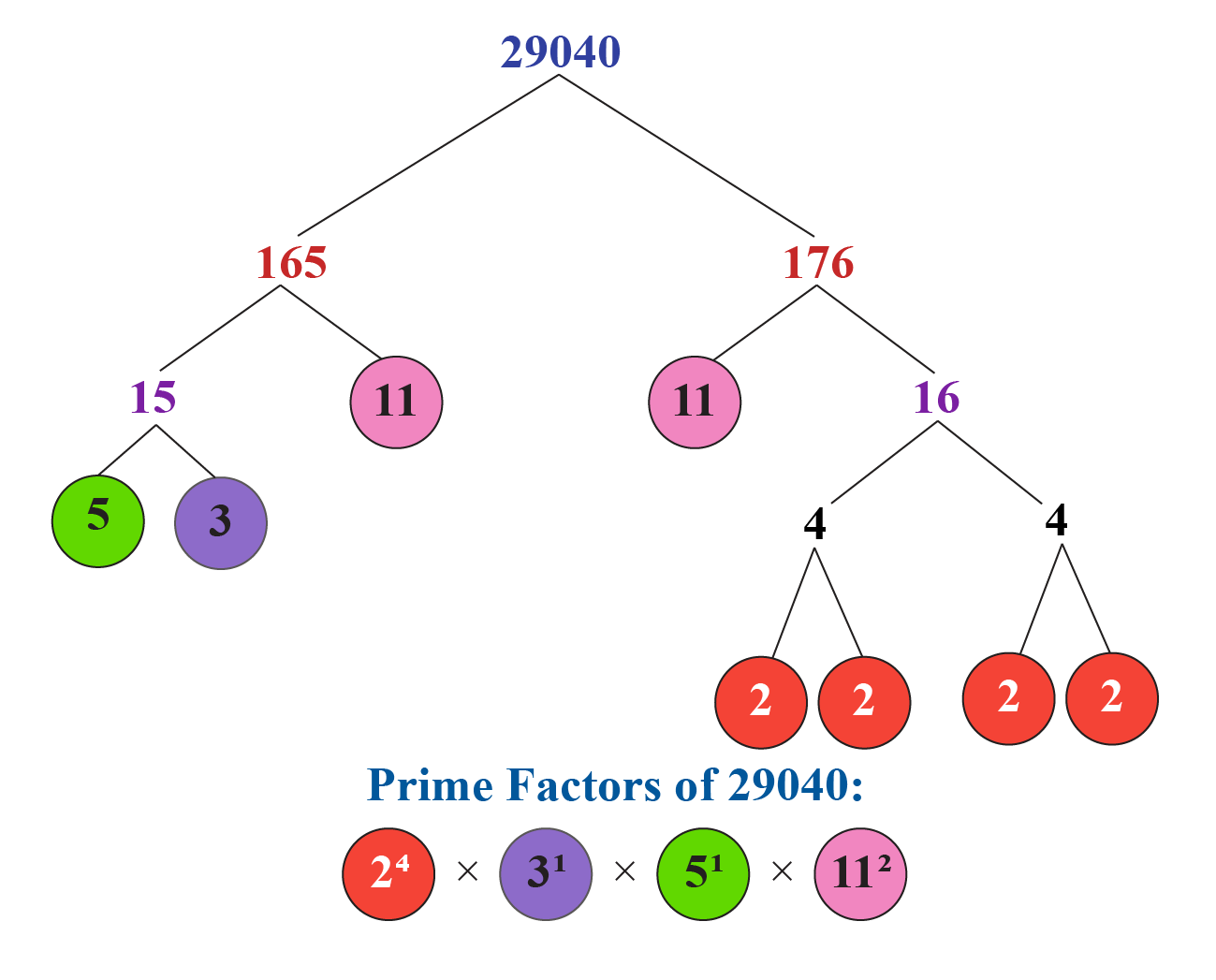The LCM will be the product of the greatest power of each prime factor occurring in the numbers:

\begin{align} {\text{LCM}}\left( {371250,\;29040} \right) &= {2^4} \times {3^3} \times {5^4} \times {11^2} \hfill \\ \qquad \qquad \qquad \qquad \quad \; &= {32670000} \hfill \\ \end{align}

 ∴ $$LCM$$ $$(371250, 29040) = 32670000$$
 Example 3

Find the LCM of the three numbers $$168, 252$$ and $$288$$ using the prime factorisation method.

Solution:

First, we carry out the prime factorization of the three numbers: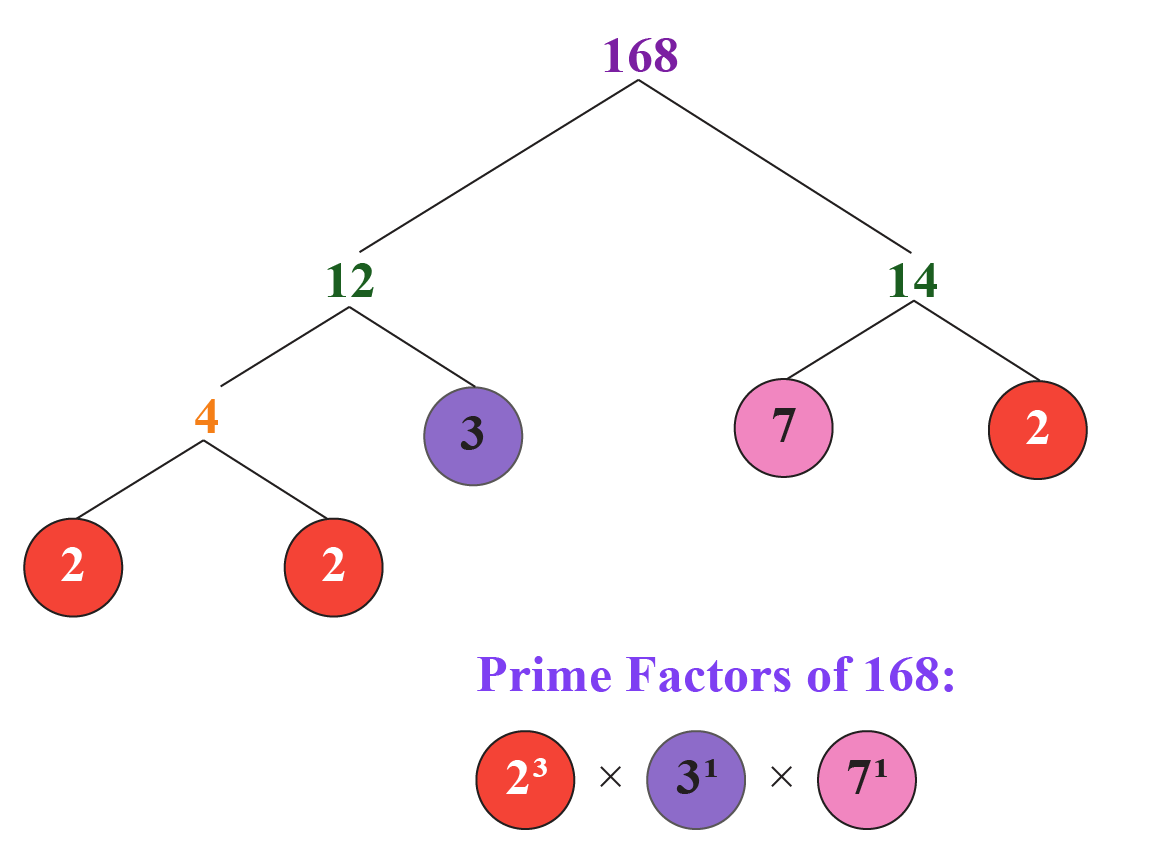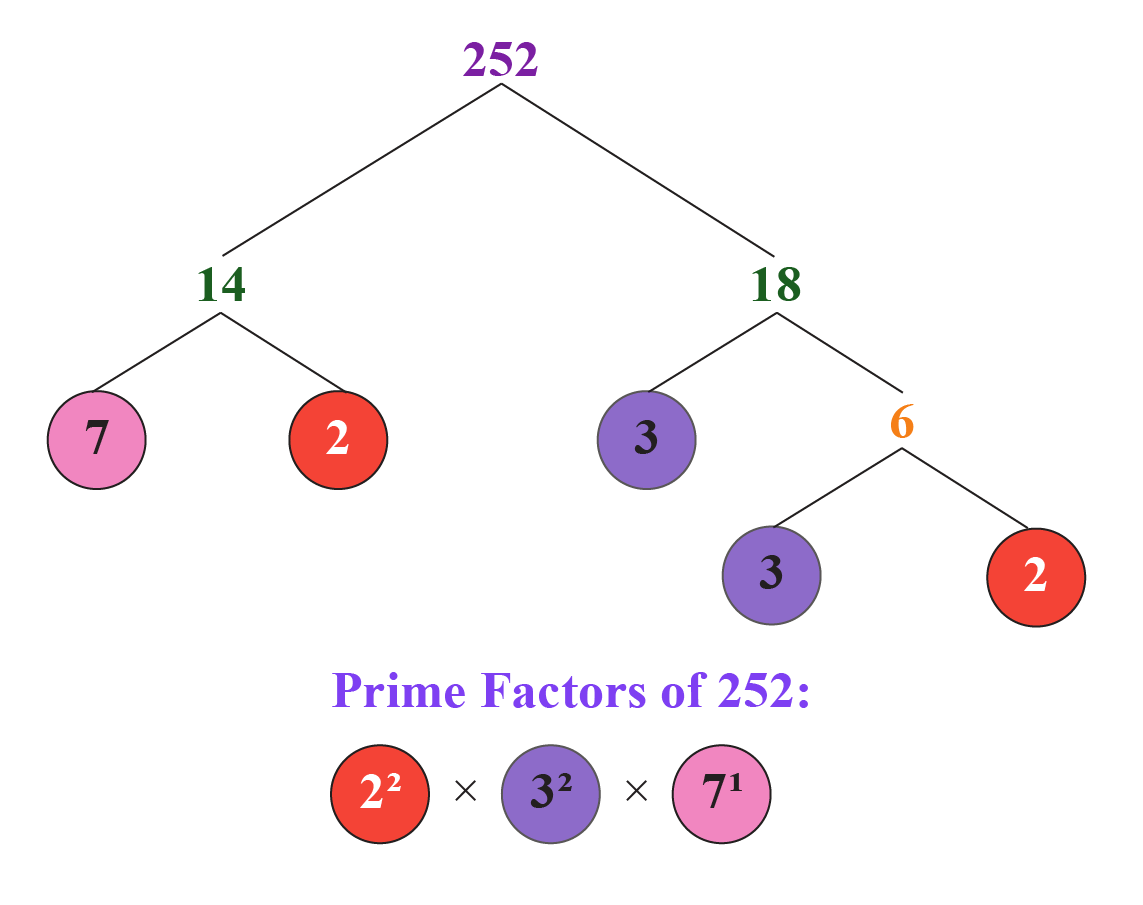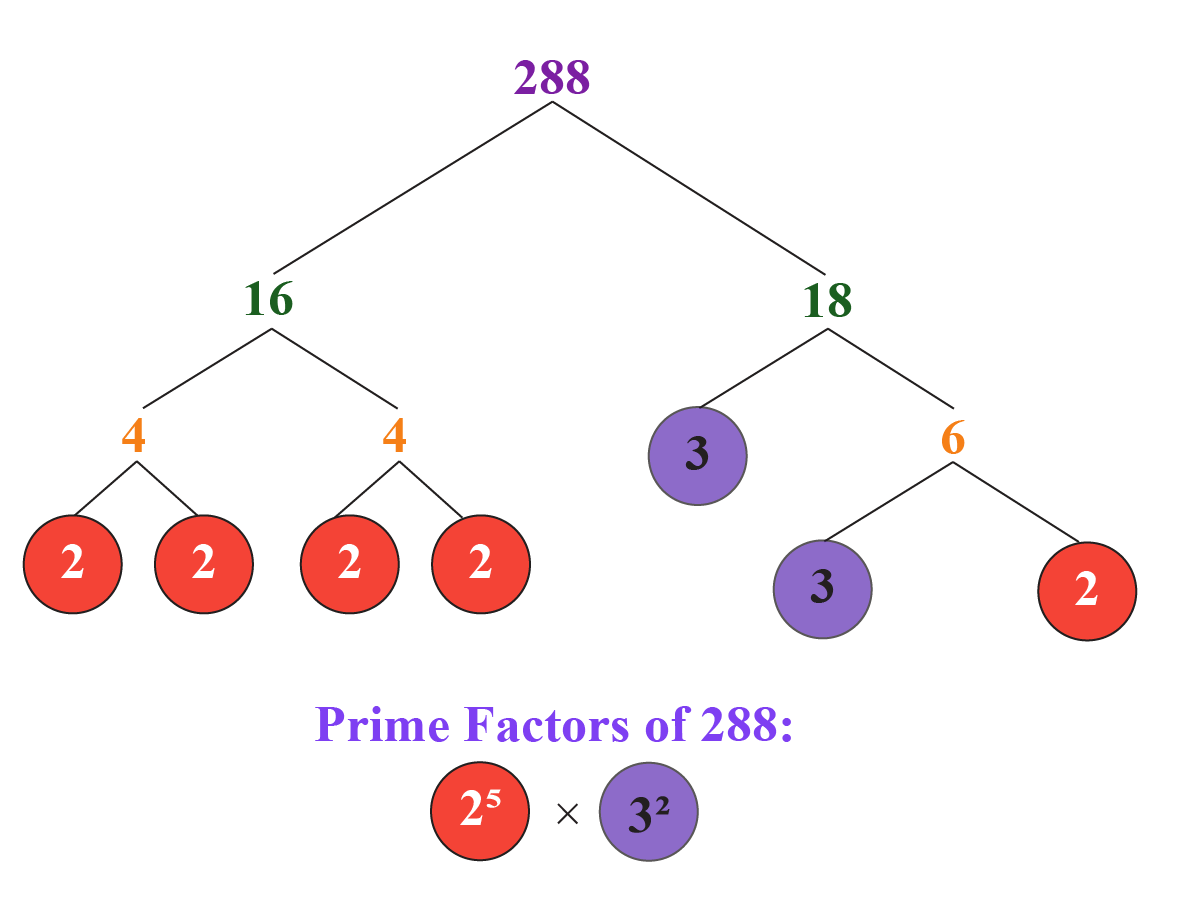The LCM will be the product of the greatest power of each prime factor occurring in the three numbers:

\begin{align} {\text{LCM}}\left( {168,\;252,\;288} \right) &= {2^5} \times {3^2} \times {7^1} \hfill \\ \qquad \qquad \qquad \qquad \quad {\text{ }} &= {2016} \hfill \\ \end{align}

 ∴ $$LCM$$ $$(168, 252, 288) = 2016$$
 Example 4

Bus X comes to a stop every 15 minutes and bus Y comes to the same stop every 40 minutes.

Let us assume that both buses are currently at the same bus stop.

After how many hours will both the buses reach the same stop again?

Solution:

To find the required time, we have to find the LCM of $$15$$ and $$40$$.

Multiples of $$15$$ are $$15, 30, 45, 60, 75, 90, 105, 120, 135, 150...$$.

Multiples of $$40$$ are $$40, 80, 120, 160,...$$.

So the LCM of $$15$$ and $$40$$ is $$120$$.

Thus both buses reach the same stop again after $$120$$ minutes which is as same as $$2$$ hours.

 ∴ $$Required$$ $$time$$ = $$2$$ $$hours$$Challenging Questions
1. Two positive numbers $$a$$ and $$b$$ are written as \begin{align} a = {x^3}{y^2} \textbf { & } b = x{y^3} \end{align} $$x$$ and $$y$$ are prime numbers. Find $${\text{LCM}}(a,b)$$.
2. Can you find the product of LCM and HCF of $$12$$ and $$18$$ without actually calculating their LCM and HCF?
3. Consider the numbers $$24$$ and $$60$$. Their HCF is $$12$$. Find their LCM.
4. Given that HCF $$(306, 657) = 9$$, find LCM $$(306, 657)$$.

## Practice Questions

Here are a few activities for you to practice. Select/Type your answer and click the "Check Answer" button to see the result.

IMO (International Maths Olympiad) is a competitive exam in Mathematics conducted annually for school students. It encourages children to develop their math solving skills from a competition perspective.

## 1. How do you find the LCM?

There are 3 methods to calculate the LCM of two numbers: by listing out the common multiples, by prime factorization method, by division method.

These methods have been explained in the previous sections with examples.

## 2. What is an example of LCM?

You can refer to the sections “Methods of Finding LCM” and “Solved Examples” sections of this page for more examples of LCM.

## 3. What is the LCM of $$4$$ and $$9$$?

Few multiples of $$4$$ are $$4, 8, 12, 16, 20, 24, 28, 32, 36, 40, …$$

Few multiples of $$9$$ are $$9, 18, 27, 36, 45, …$$

The LCM of $$4$$ and $$9$$ is the smallest multiple that is a common multiple of both of the numbers.

Thus, the LCM of $$4$$ and $$9$$ is $$36$$.

## 4. What is the LCM of $$8$$ and $$12$$?

Few multiples of $$8$$ are $$8, 16, 24, 32, …$$.

Few multiples of $$12$$ are $$12, 24, 36, 48 …$$.

The LCM of $$8$$ and $$12$$ is the smallest number among all common multiples of both of the numbers.

Thus, the LCM of $$8$$ and $$12$$ is $$24$$.

## 5. What is LCM in math?

The least common multiple of two or more numbers is the smallest number among all common multiples of the given numbers.

Arithmetic Integers
grade 10 | Questions Set 1
Arithmetic Integers
Arithmetic Integers
grade 10 | Questions Set 2
Arithmetic Integers
HCF and LCM